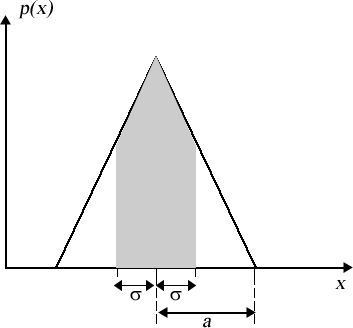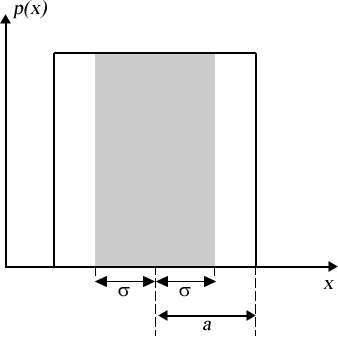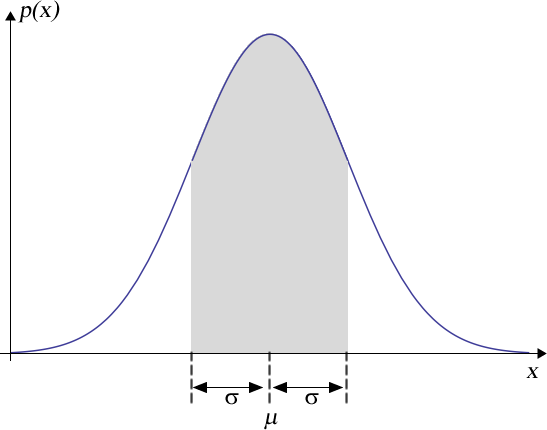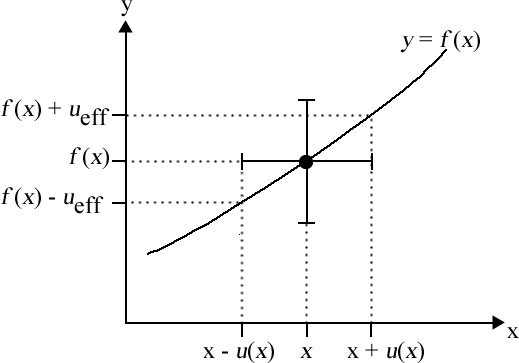# Uncertainty in Physical Measurements: Summary

Uncertainty Module 7 - Summary Student Guide May 6, 2017, 1:30 p.m.

Here we summarise some of the materials we learned about in the previous Modules.

# Probability Distribution Functions

A key concept is a probability distribution function (pdf). Some sources call it a probability density function. It represents the probability that some quantity that is being measured takes on a particular value. Calling the quantity that is being measured x we write the pdf as p(x). Since the quantity x that is being measured always has some value, the total area under the pdf is exactly equal to 1.

For a symmetric pdf, the centre value has the highest probability. It is the best approximation of the value of the measurand. The width of a pdf is characterized by its standard deviation $$\sigma$$. Its value depends on the shape of the pdf. If the centre value of the pdf is xc, then the area under the pdf between $$x_c - \sigma$$ and $$x_c + \sigma$$ has a probability whose value depends on the particular pdf but is always less than 1. Thus, the true value of the measurand probably lies between  $$x_c - \sigma$$  and  $$x_c + \sigma$$ . Therefore, the standard deviation is the standard uncertainty u(x) of the value of the measurand.

Here are the probability distribution functions that we usually use for different types of measurements.

 Type of Measurement Probability Distribution Function Standard Deviation Shaded Area Analog Triangular$$\sigma = \frac{a}{{\sqrt 6 }}$$ 0.65 Digital Rectangular or Uniform$$\sigma = \frac{a}{{\sqrt 3 }}$$ 0.58 N Repeated Measurements Gaussian or Bell-Shaped or Normal Distribution$$\sigma _{est} = \sqrt {\frac{{\sum\limits_{i = 1}^N {{{\left( {{x_i} - {{\bar x}_{est}}} \right)}^2}} }}{{N - 1}}}$$ where: $$\bar{x}_{est} = \frac{{\sum\limits_{i = 1}^N {{x_i}} }}{N}$$ $$u(\bar{x}_{est}) = \sigma / \sqrt{N}$$ 0.68

# Propagation of Uncertainties

If z = x + y or z = xy then the uncertainties are combined in quadrature:

$$u(z) = \sqrt{ u(x)^2 + u(y)^2 }$$

## Multiplication or Division

If $$z = x \times y$$  or $$z = x \div y$$  then the fractional uncertainties are combined in quadrature:

$$\frac{{u(z)}}{z} = \sqrt {{{\left( {\frac{{u(x)}}{x}} \right)}^2} + {{\left( {\frac{{u(y)}}{y}} \right)}^2}}$$

## Multiplication by a Constant

If  $$z = a \times x$$  where a is a constant known to a large number of significant figures, then the uncertainty in z is given by:

$$u(z) = a u(x)$$

## Raising to a Power

If  $$z = x^n$$ then:

$$u(z) = n x^{(n - 1)} u(x)$$

which can also be written in terms of the fractional uncertainties:

$$\frac{{u(z)}}{z} = n\frac{{u\left( x \right)}}{x}$$

## The General Case

If z = f(x, y) then:

$$u(z) = \sqrt {{{\left( {\frac{{\partial f(x,y)}}{{\partial x}}u(x)} \right)}^2} + {{\left( {\frac{{\partial f(x,y)}}{{\partial y}}u(y)} \right)}^2}}$$

# Least-Squares Fitting

In general we fit to a model, y = f(x). The dependent variable is y, and the independent variable is x.

The degrees of freedom of the fit is the number of parameters to which we are fitting minus the number of datapoints.

For each datapoint, the residual is:

$$r_i = y_i - f(x_i)$$

## Data Without Explicit Uncertainties

The sum of the squares of the residuals is:

$$ss = \sum\limits_{i = 1}^N {{{\left( {{r_i}} \right)}^2}}$$

The fit finds values of the fitted parameters for which the sum of the squares of the residuals is a minimum. The value of ss is a measure of the quality of the fit.

## Data With Explicit Uncertainties Only in the Dependent Variable

The chi-squared is:

$${\chi ^2} = {\sum\limits_{i = 1}^N {\left[ {\frac{{{r_i}}}{{u({y_i})}}} \right]} ^2}\$$

The fit finds values of the fitted parameters for which the $$\chi^2$$ is a minimum.

For good data fit to an appropriate model, the $$\chi^2$$ should be roughly equal to the degrees of freedom.

## Data With Explicit Uncertainties in Both VariablesThe effective variance method finds the effective uncertainty in y, ueff, due to the uncertainty in x.

Then the effective uncertainty in y is: $${u_{total}}(y) = \sqrt {u{{(y)}^2} + {u_{eff}}^2}$$

This uncertainty is used just as u(h) is for the case of a data with an explicit uncertainty only in the dependent variable. The chi-squared is interpreted just as for that case.

# Uncertainty in an Integer Count

If we count N things, a good first guess of the statistical uncertainty in the value of N is $$u(N) = \sqrt{N}$$ .

# Systematic Errors

Systematic errors are biases in a measurement that cause the result to be systematically incorrect.

Sometimes the biases are indicative of an unknown physical phenomenon.

Sometimes the biases are because of problems with the instrument or the way the experimenter reads it. Calibration can sometimes correct for these biases.

# Robust Estimators

A robust estimator is a value that is not greatly influenced by one or more outliers in a dataset.

Quartiles are three points that divide a dataset into four equal groups, each containing one-quarter of the data. They are robust.

The first quartile splits off the lowest 25% of the data from the highest 75%.

The second quartile divides the data into two equal sized groups. It is also called the median.

The third quartile splits off the highest 25% of the data from the lowest 75%.

The interquartile range (IQR) is the difference between the third quartile and the first quartile.

The median and its uncertainty are given by:

$${\text{median}} \pm \frac{ 1.58 \times{{\text{IQR}}}}{{\sqrt N }}$$

This Summary was written by David M. Harrison, Dept. of Physics, Univ. of Toronto, October 2013